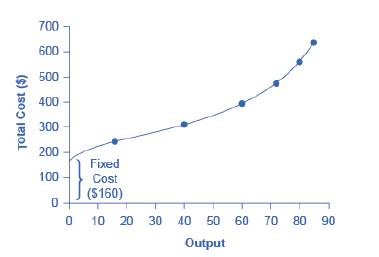Chapter 7, Problem 40P### Principles of Economics 2e

2nd Edition
Steven A. Greenlaw; David Shapiro
ISBN: 9781947172364

#### Solutions

Chapter
Section### Principles of Economics 2e

2nd Edition
Steven A. Greenlaw; David Shapiro
ISBN: 9781947172364
Textbook Problem

# Return to Figure 7.7. What is the marginal gain in output from increasing the number of batters from 4 to 5 and from 5 to 6? Does it continue the pattern of diminishing marginal returns?Figure 7.7 How output Affects Total costs

To determine

The marginal gain in output due to the increase in the number of barbers and to evaluate its effect on the pattern of diminishing marginal returns.

Explanation

Due to the increase in the number of barbers from 4 to 5, the marginal gain will be calculated as the difference in the quantities of the given unit of output. It can be seen as below:

=8072=8

If the number of barbers increases from 5 to 6, the marginal gain will be calculated as the difference in the quantities of the given unit of output

### Still sussing out bartleby?

Check out a sample textbook solution.

See a sample solution

#### The Solution to Your Study Problems

Bartleby provides explanations to thousands of textbook problems written by our experts, many with advanced degrees!

Get Started

#### Find more solutions based on key concepts